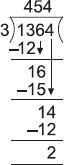# User Forum

Subject :IMO    Class : Class 4

Kartik solved the division problem as shown. Which expression could be used to check his answer?A (454 × 3) + 2
B (454 × 3) + 3
C (454 × 3) × 2
D (454 × 3) × 3

## Ans 1:

Class : Class 8
The answer is A because the formula to check the answer in division is - Dividend = (Quotient X Divisor) Remainder. So, it will be 1364= (454 X 3) 2

Class : Class 5
a

Class : Class 4
A.